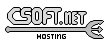<-- Back to AG_Intro.3

# SYNOPSIS

 ```#include #include #include ```

# DESCRIPTION

The M_PointSet family of structures describe arbitrary sets of points in space. They are defined as follows:
 ```/* Points in R2 */ typedef struct m_point_set2 { M_Vector2 *p; Uint n, nMax; } M_PointSet2; /* Points in Z^2 */ typedef struct m_point_set2i { M_Real w, h; int *x, *y; Uint n, nMax; } M_PointSet2i; /* Points in R3 */ typedef struct m_point_set3 { M_Vector3 *p; Uint n, nMax; } M_PointSet3; /* Points in Z^3 */ typedef struct m_point_set3i { M_Real w, h, d; /* Scaling factor */ int *x, *y, *z; Uint n, nMax; } M_PointSet3i; ```

The points in a M_PointSet are stored under the p array of vectors (see M_Vector(3)).

The points in M_PointSeti are stored as separate arrays of integers x, y, z. The w, h and d members specify scaling factors to use when converting from an integer set to a real set.

# INITIALIZATION

 void M_PointSetInit2 (M_PointSet2 *S)void M_PointSetInit3 (M_PointSet3 *S)void M_PointSetInit2i (M_PointSet2i *S, M_Real w, M_Real h)void M_PointSetInit3i (M_PointSet3i *S, M_Real w, M_Real h, M_Real d)int M_PointSetAlloc2 (M_PointSet2 *S, Uint n)int M_PointSetAlloc3 (M_PointSet3 *S, Uint n)int M_PointSetAlloc2i (M_PointSet2i *S, Uint n)int M_PointSetAlloc3i (M_PointSet3i *S, Uint n)void M_PointSetFree2 (M_PointSet2 *S)void M_PointSetFree3 (M_PointSet3 *S)void M_PointSetFree2i (M_PointSet2i *S)void M_PointSetFree3i (M_PointSet2i *S)M_PointSet2 M_PointSetRead2 (AG_DataSource *ds)void M_PointSetWrite2 (AG_DataSource *ds, const M_PointSet2 *S)M_PointSet3 M_PointSetRead3 (AG_DataSource *ds)void M_PointSetWrite3 (AG_DataSource *ds, const M_PointSet3 *S)M_PointSet2i M_PointSetRead2i (AG_DataSource *ds)void M_PointSetWrite2i (AG_DataSource *ds, const M_PointSet2i *S)M_PointSet3i M_PointSetRead3i (AG_DataSource *ds)void M_PointSetWrite3i (AG_DataSource *ds, const M_PointSet3i *S)M_PointSet2 M_POINT_SET2_EMPTY (void)M_PointSet3 M_POINT_SET3_EMPTY (void)M_PointSet2i M_POINT_SET2I_EMPTY (void)M_PointSet3i M_POINT_SET3I_EMPTY (void)

The M_PointSetInit() functions initialize a point set in R2 or R3 to the null set.

The M_PointSetIniti() functions initialize a point set in Z^3 or Z^3 to the null set. The w, h, d arguments specify the scaling factor to use when converting from an integer to a real point set.

The M_PointSetAlloc*() functions allocates memory for the specified number of points, returning 0 on success or -1 if insufficient memory is available.

The M_PointSetFree*() functions free the point sets, clearing the arrays and reinitializing the point count to 0.

The M_PointSetRead*() and M_TriangleWrite*() functions read or write a triangle structure from/to an AG_DataSource(3).

The macros M_POINT_SET*_EMPTY() expand to static initializers for any of the M_PointSet structures.

# OPERATIONS

 int M_PointSetAdd2 (M_PointSet2 *S, M_Vector2 v)int M_PointSetAdd3 (M_PointSet3 *S, M_Vector3 v)int M_PointSetAdd2i (M_PointSet2 *S, int x, int y)int M_PointSetAdd3i (M_PointSet3i *S, int x, int y, int z)int M_PointSetCopy2 (M_PointSet2 *D, const M_PointSet2 *S)int M_PointSetCopy3 (M_PointSet3 *D, const M_PointSet3 *S)int M_PointSetCopy2i (M_PointSet2i *D, const M_PointSet2i *S)int M_PointSetCopy3i (M_PointSet3i *D, const M_PointSet3i *S)void M_PointSetSort2 (M_PointSet2 *S, enum m_point_set_sort_mode2)void M_PointSetSort3 (M_PointSet3 *S, enum m_point_set_sort_mode3)

The M_PointSetAdd*() functions insert a new point at the end of the set S. On success, the index of the new point is returned. If insufficient memory is available, -1 is returned.

The M_PointSetCopy*() functions copy the contents of source set S into destination set D, returning 0 on success or -1 if insufficient memory is available.

The M_PointSetSort() functions sort the point sets by point coordinate. The mode arguments specify the sorting mode:
 ```enum m_point_set_sort_mode2 { M_POINT_SET_SORT_XY, M_POINT_SET_SORT_YX, }; enum m_point_set_sort_mode3 { M_POINT_SET_SORT_XYZ, M_POINT_SET_SORT_XZY, M_POINT_SET_SORT_YXZ, M_POINT_SET_SORT_YZX, M_POINT_SET_SORT_ZXY, M_POINT_SET_SORT_ZYX, }; ```